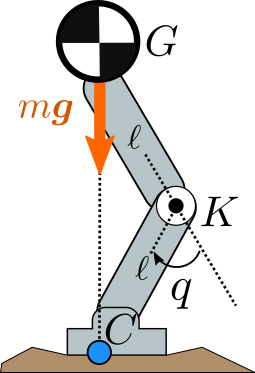# Knee torque of a lumped mass modelConsider the planar legged model depicted to the right. The robot's mass is concentrated at its center of mass $$G$$, meaning we neglect the mass of the two links of the leg. The center of mass is located above the center of pressure $$C$$ and the robot is in static equilibrium. The leg has a single revolute joint located at the knee $$K$$ with joint angle $$q$$. Both of its links have the same length $$CK = KG = \ell$$.

Question: what is the joint torque $$\tau$$ exerted by the knee to keep the leg in static equilibrium?

## Note

This is a question page: there is no need to post the answer in the discussion below. However, if you have an original derivation of the solution, you are welcome to post it.

## To go further

This simplified model was considered to dimension the knee actuators during the design of the HRP-4 humanoid robot.

## Discussion

You can use Markdown with $\LaTeX$ formulas in your comment.